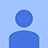﻿Đề thi Violympic Toán Tiếng Anh lớp 8 vòng 10 năm 2014 - 2015 - Đề thi giải Toán tiếng Anh qua mạng lớp 8 cấp quốc gia - VnDoc.com

# Đề thi Violympic Toán Tiếng Anh lớp 8 vòng 10 năm 2014 - 2015

63 4.428

## Đề thi giải Toán tiếng Anh qua mạng lớp 8 cấp quốc gia

Vòng 10 (vòng thi cấp quốc gia) của Cuộc thi giải Toán Tiếng Anh qua mạng năm 2015 - 2016 bắt đầu mở từ ngày 08/04/2016. Để ôn tập và chuẩn bị cho vòng thi này mời các bạn tham khảo bài test Đề thi Violympic Toán Tiếng Anh lớp 8 vòng 10 năm 2014 - 2015 trên trang VnDoc.com. Tham gia làm bài test để mở rộng vốn từ vựng tiếng anh về toán học đồng thời nâng cao kỹ năng giải toán nhé!

• Exam number 1: Fill in the blank with the suitable number
Question 1:
When the polynomial f(x) = 3x3 + 2x2 + 5x + 1 is divided by g(x) = x + 1, the remainder is ............
-5
• Question 2:
If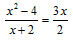then x = .............
-4
• Question 3:

Given that 75k is perfect cube, the least positive integer k is ...........

45
• Question 4:

If n is a natural number such that n2 - 5 is a square number, then n = .......

3
• Question 5:
On the number line, point A has coordinate -4 and point B has coordinate 6. If point C is the midpoint of AB, then its coordinate is .............
1
• Question 6:
The root of the equation Ιx2 - 1Ι = x - 1 is x = .........
1
• Question 7:
If the sum of al interior angles in a convex polygon is 1080 degrees, then this polygon has ........... diagonals.
20
• Question 8:
A triangle ABC has Â = 120o and the bisector AD (D  BC). If AB = 40cm, AD = 30cm, then AC = ..... cm.
120
• Question 9:

Given two numbers x, y such that (4y2 - 12y + 25)(4x2 + 6x + 4) = 28
The ratio of y to x is ..........

-2
• Question 10:
Mr.Joseph drives car from A to B at a constant speed. If the speed of the car is increased by 20%, it takes him one hour less than the usual time. If he drives at the constant speed for the first 100km before increasing the speed by 30%, it also takes him one hour less than the usual. The distance of AB is ..........km.
360
• Exam number 2: Choose the best answer.
Question 1:
The perimeters of two similar triangles ABC, DEF are 36cm and 24cm respectively. If DE = 10cm, then AB = ...... cm.
• Question 2:
Find the value of the constant m such that the polynomial f(x) = x3 - x + m is divisible by x + 2.
• Question 3:
If x = 3; y = -1 then x5 + 5x4y + 10x3y2 + 10x2y3 + 5xy4 + y5 = .................
• Question 4:

Which of the following statements is NOT true?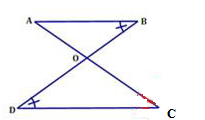• Question 5:
As shown in the figure, the length of BE is .............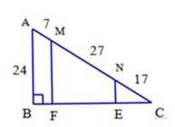• Question 6:

On a car trip Sam drove m miles, Kara drove twice as many miles as Sam did and Darin drove 20 miles less than Kara did. In terms of m, how many miles did Darin drive?

• Question 7:
Given that two numbers x and y satisfy 2 < x < 3 and 7 < y < 8. Which of the following expressions has the greatest value?
• Question 8:
The solution set of the equation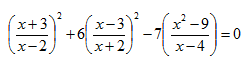is S = {........}
• Question 9:

Let f(x) the polynomial given by f(x) = (1 + 2x + 3x2 + 4x3 + 5x4 + 84x5)
Suppose that f(x) = ao + a1x + a2x+ ..... + ..... + a50x50.
The value of T = a+ a+ .... + a50 is .........

• Question 10:
The maximum value of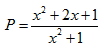is ...........
• Exam number 3:
Question 1:
One rectangle with the sides x and 3x. One isosceles right triangle with the length of leg x. What is the ratio of the area of the reactangle to that of the triangle?
6
• Question 2:

Given the function f(x) = 1/4 .m(m + 2)x2 + 3/2. mx + 3 with f(2) = -3.
The greatest possible value of m is .........

-2
• Question 3:
If a + 2b = 6 and ab = 4 then 4/a + 2/b = ......
3
• Question 4:
Let S(x; y) = x2 - y2 with real numbers x and y, then S(3; S(3; 4)) = ...........
-40
• Quesstion 5:
Given a rectangular land such that its length is twice as its width. If the width is decreased by 5m and the length is increased by 12m then the area is increased by 10 square meters. The width of this land is ........ m.
35
• Question 6:

In the xy - plane, given three points A(-1; 2); B(-1; 8); C(5; 8). If ABCD is a square then the coordinate of D is (.....).  Write your answer with the semicolon between x- coordinate and y- coordinate.

(5; 2)
• Question 7:
The route AB is 60km long. At 7a.m, one motorbike travels from A to B with a constant speed. When this motorbike reaches B, it returns to A immediately with the speed increased by 10km/h and reaches A at 10.30 a.m. The original speed of this motorbike is ........ km/h.
30
• Question 8:
Find the value of n such that A = n3 - 2n2 + 2n - 4 is a prime number. The value of n is ..........
3
• Question 9:

Find the area of the trapezoid ABCD, BC // AD, AB = CD = 5cm, BC = 10cm, AD = 16cm.
The area of the trapezoid ABCD is .........cm2.

52
• Question 10:
Find the least possible value of A = 4x2 - 3x + 1/4x + 2015, where x varies in the set of positive real numbers. The least possible value of A is ..........
2015
• Đáp án đúng của hệ thống
• Trả lời đúng của bạn
• Trả lời sai của bạn
Đánh giá bài viết
63 4.428
Sắp xếp theo